Latest Banking jobs   »

# Quantitative Aptitude Quiz For RBI Grade B/ ECGC PO/ SIDBI Grade A Prelims 2022- 14th May

Q1. Veer is 40% less efficient than Bhavya and both complete the work together in 30 days, if Abhishek takes 10 days less as Veer and Bhavya takes together. Then find how many days will be required to complete the work, if all three work together?
(a) 16 days
(b) 12 days
(c) 10 days
(d) 8 days
(e) 18 days

Q2. Sameer borrowed 17500 Rs. from Divyaraj on compound interest annually at the rate of 20% per annum, if he paid 5000 Rs. at the end of every year to Divyaraj then find how much amount Sameer have to pay at the end of fourth year for complete his debt?
(a) 14168
(b) 14648
(c) 14848
(d) 14448
(e) 14248

Q3. Ankit gets 6000 Rs. out of total profit of 9000 Rs. and he invested 8000 more amount than his partner for 8 months and his partner Arun invested his amount for whole the year in partnership, then find how much amount invested by Ankit?
(a) 8000 Rs.
(b) 1000 Rs.
(c) 9000 Rs.
(d) 14000 Rs.
(e) 12000 Rs.

Q4. Cost price of a table is 35% more than cost price of a chair, if shopkeeper sold chair at 25% profit and table at 33⅓% of loss he gets the total loss of 1350 Rs. find the cost price of table?
(a) 9012.5 Rs.
(b) 9112.5 Rs.
(c) 9212.5 Rs.
(d) 9002.5 Rs.
(e) 9122.5 Rs.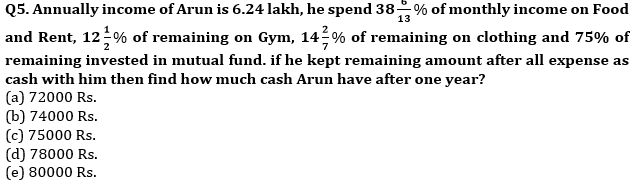Directions (6-10): Each of the following question is followed by two statements I, and II. You have to study the question and the statements and decide which of the statement(s) is/are necessary to answer the question.
(a) Only Statement I alone.
(b) Only Statement II alone.
(c) Both Statements I and II together.
(d) Neither Statement I nor II is sufficient.
(e) Either Statement I or II alone.

Q6. What is the speed of train (in kmph)?
Statement I: the train crosses a person walking in same direction in 10 sec.
Statement II: the train crosses a 200 m long platform in 20 sec.

Q7. What is the probability of drawing 2 red balls?
Statement I: there are 4 red and 5 blue balls in the bag.
Statement II: there are 20 balls in the bag out of which 5 are yellow and rest are blue & red.

Q8. In how many days 12 men can finish the work?
Statement I: 15 women can complete the same work in 20 days.
Statement II: A man is 20% more efficient than a woman.

Q9. What is the rate of interest (per annum)?
Statement I: Rs. 2000 fetch an interest of Rs. 400.
Statement II: Rs. 2000 becomes Rs. 2420 in 2 years when invested at compound interest compounding annually.

Q10. What is the average age of family?
Statement I: The age of Father is twice age of son who is 4 years elder to his sister whose age is half of her mother.
Statement II: The age of oldest person is 40 years and there are only 4 persons in the family.

Directions (11-15): In the following questions, two equations (I) and (II) are given. You have to solve both the equations and answer the following questions.
(a) x<y
(b) x>y
(c) x≤y
(d) x≥y
(e) x=y or no relation.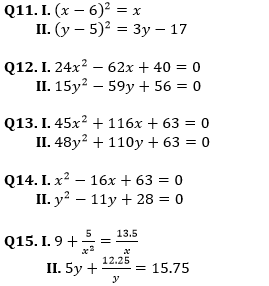Solutions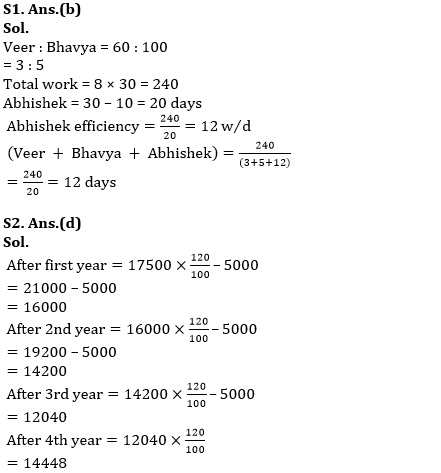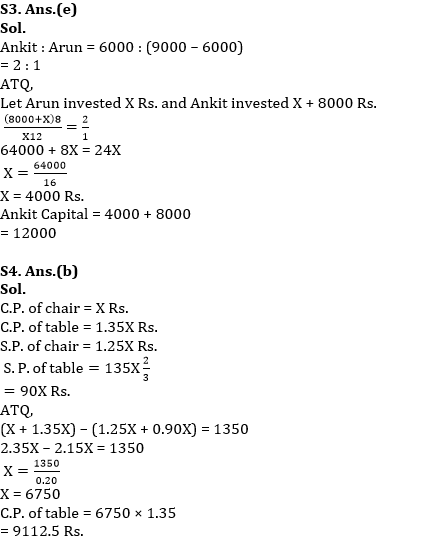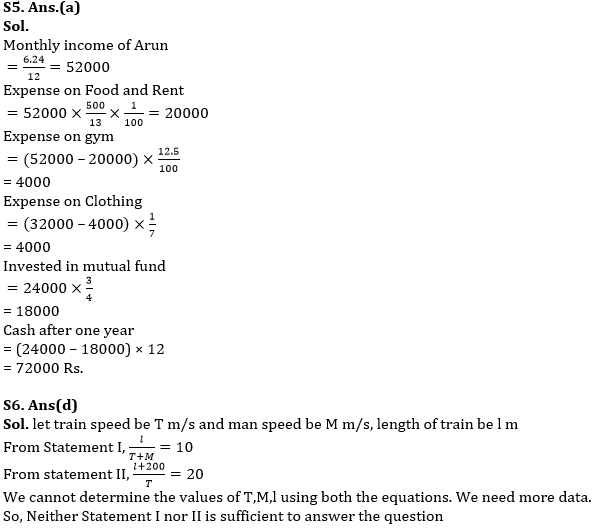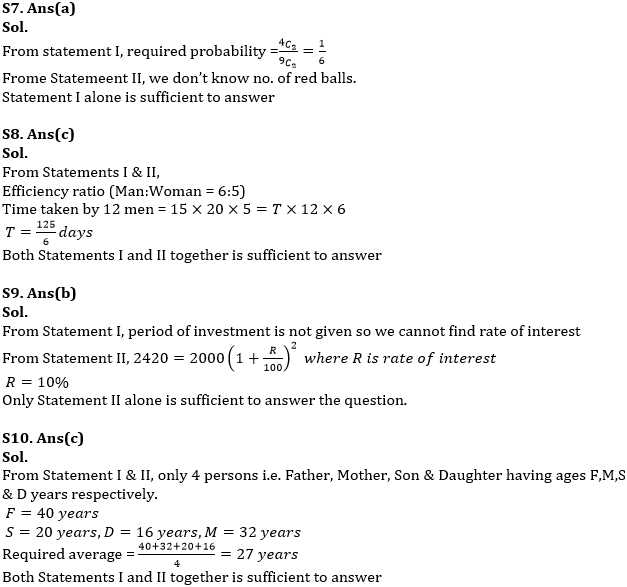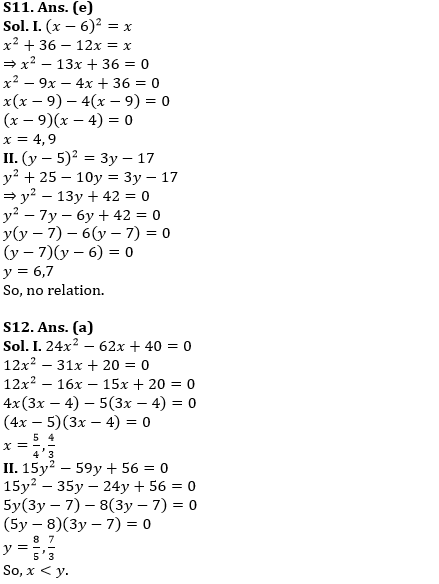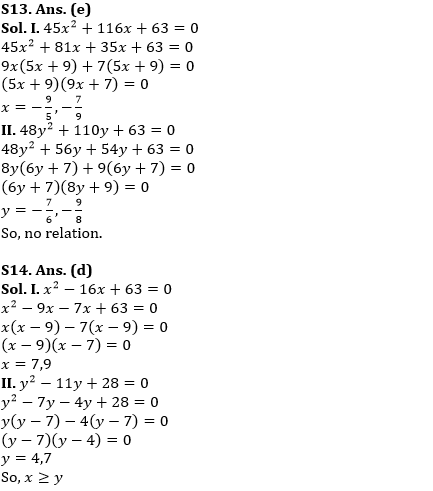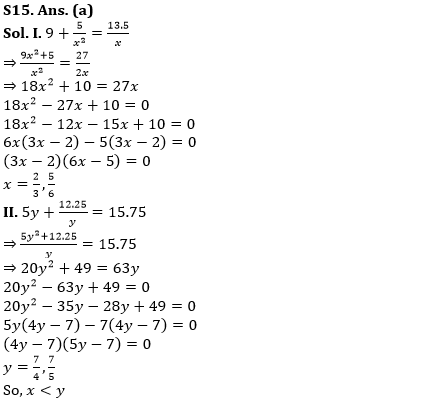#### Congratulations!Union Budget 2023-24: Free PDF